# RS Aggarwal Solutions for Class 6 Maths Chapter 9 Linear Equations in One Variable

## Class 6 RS Aggarwal Chapter 9 – Linear Equations in One Variable

RS Aggarwal Solutions for Class 6 Maths Chapter 9 Linear Equations in One Variable are provided here. Students are advised to go through the RS Aggarwal Solutions for Class 6 Maths to prepare well for the exam and to gain high marks. Practising RS Aggarwal Solutions diligently help students to score high marks in the annual exam. By going through RS Aggarwal Solutions, students will clearly understand the Chapter. Practising textbook questions will help the students in boosting their self- confidence and to understand the topics discussed in this Chapter in detail.

Students are advised to go through the RS Aggarwal Solutions for Class 6 Chapter 9 which have been solved by the BYJU’S experts in pdf format. Download pdf of Class 6 Chapter 9 in their respective links.

## Download PDF of RS Aggarwal Solutions for Class 6 Maths Chapter 9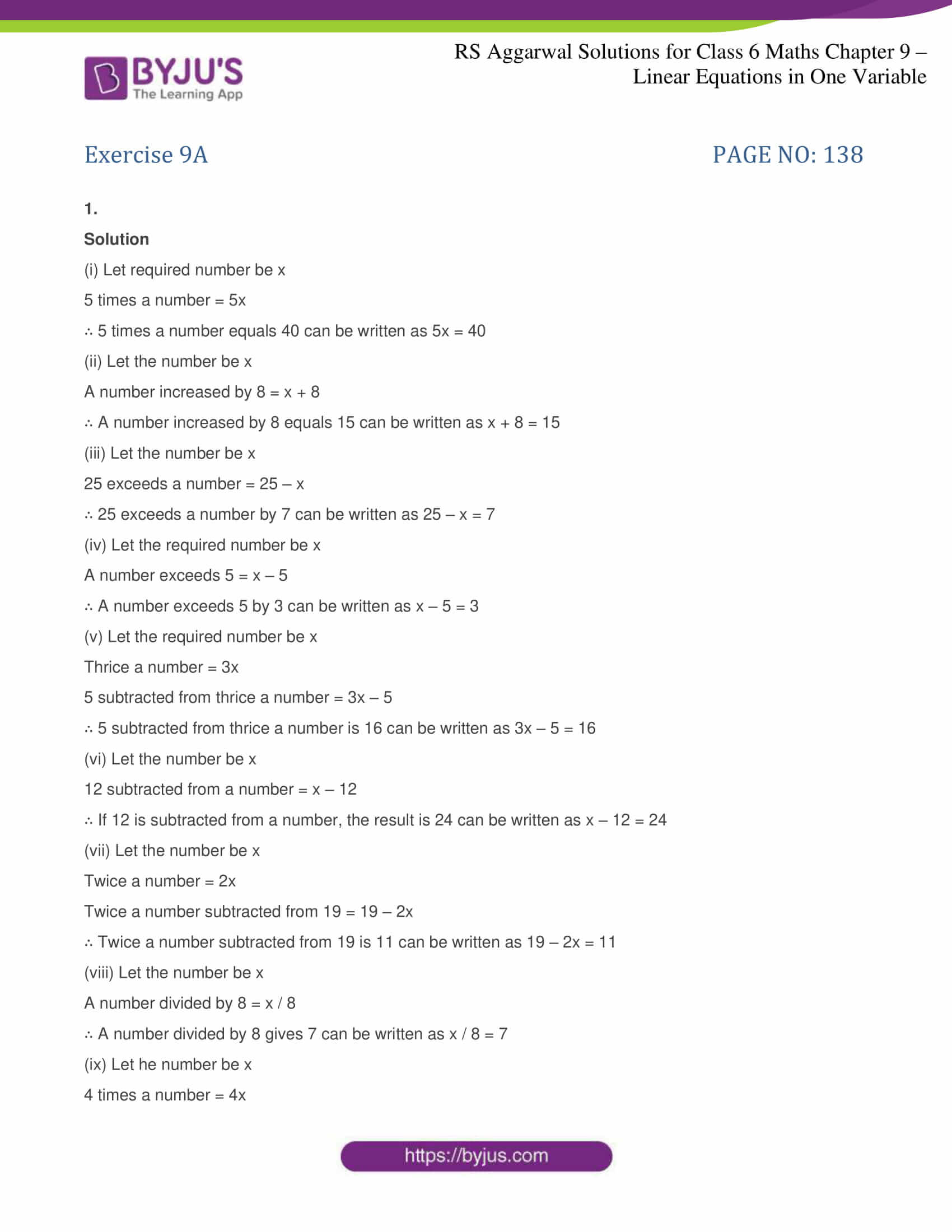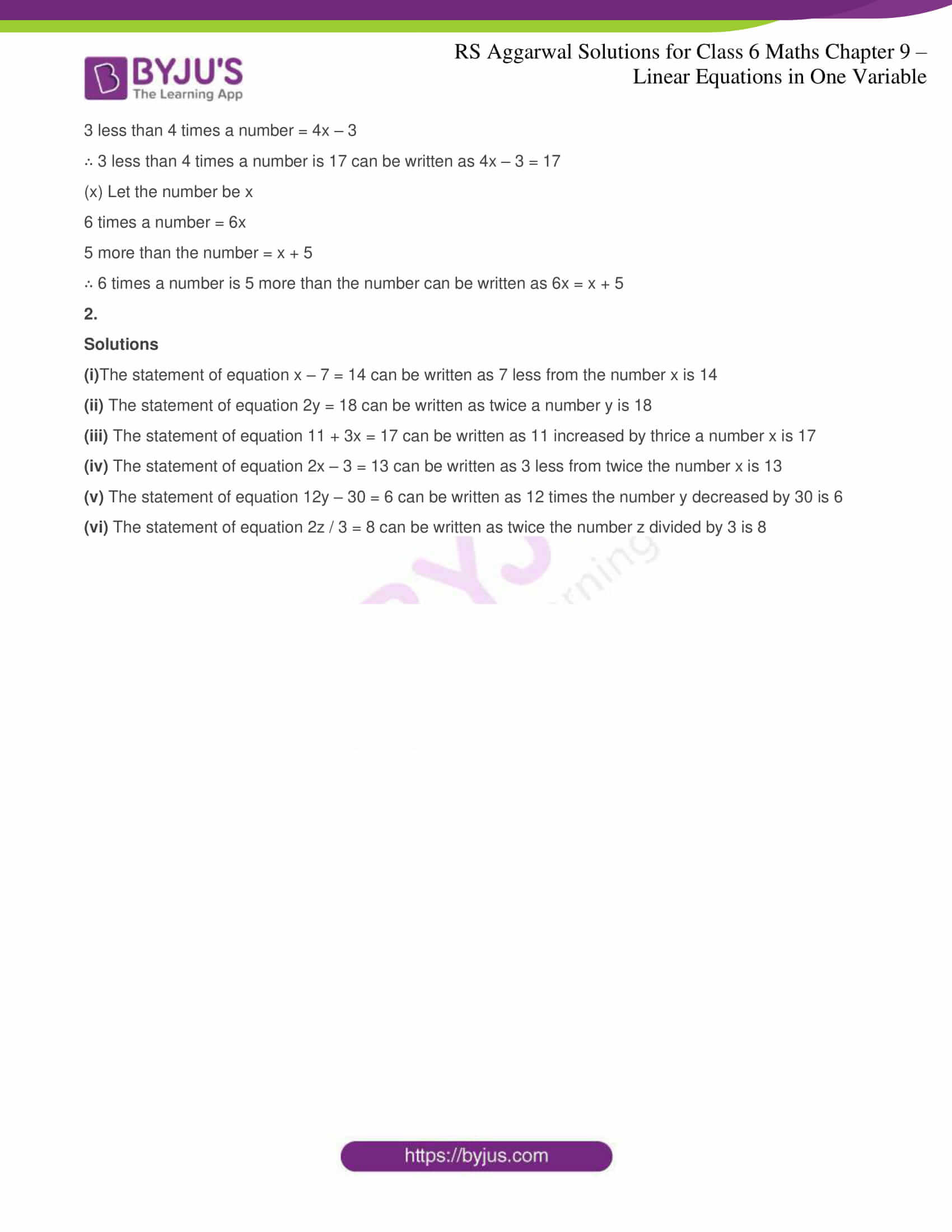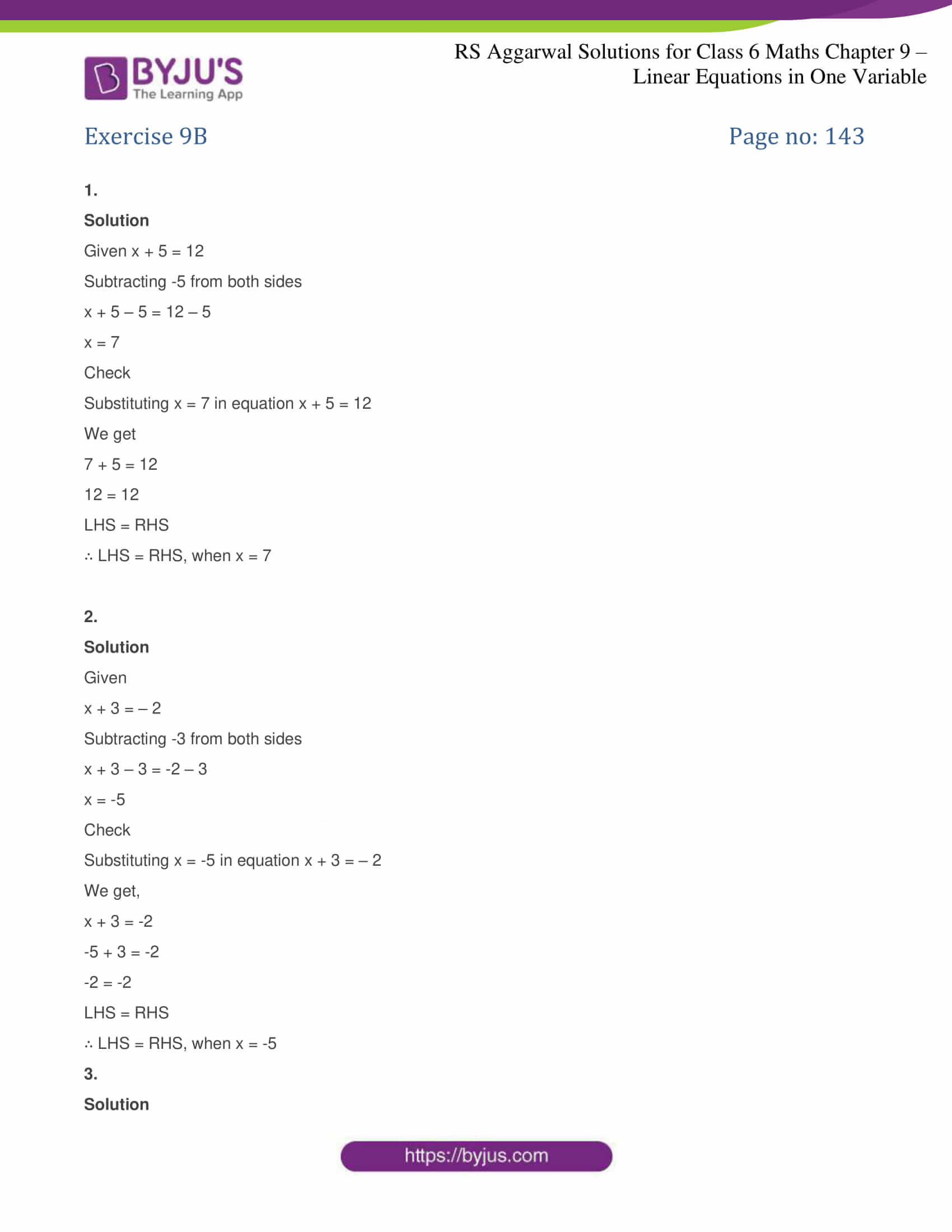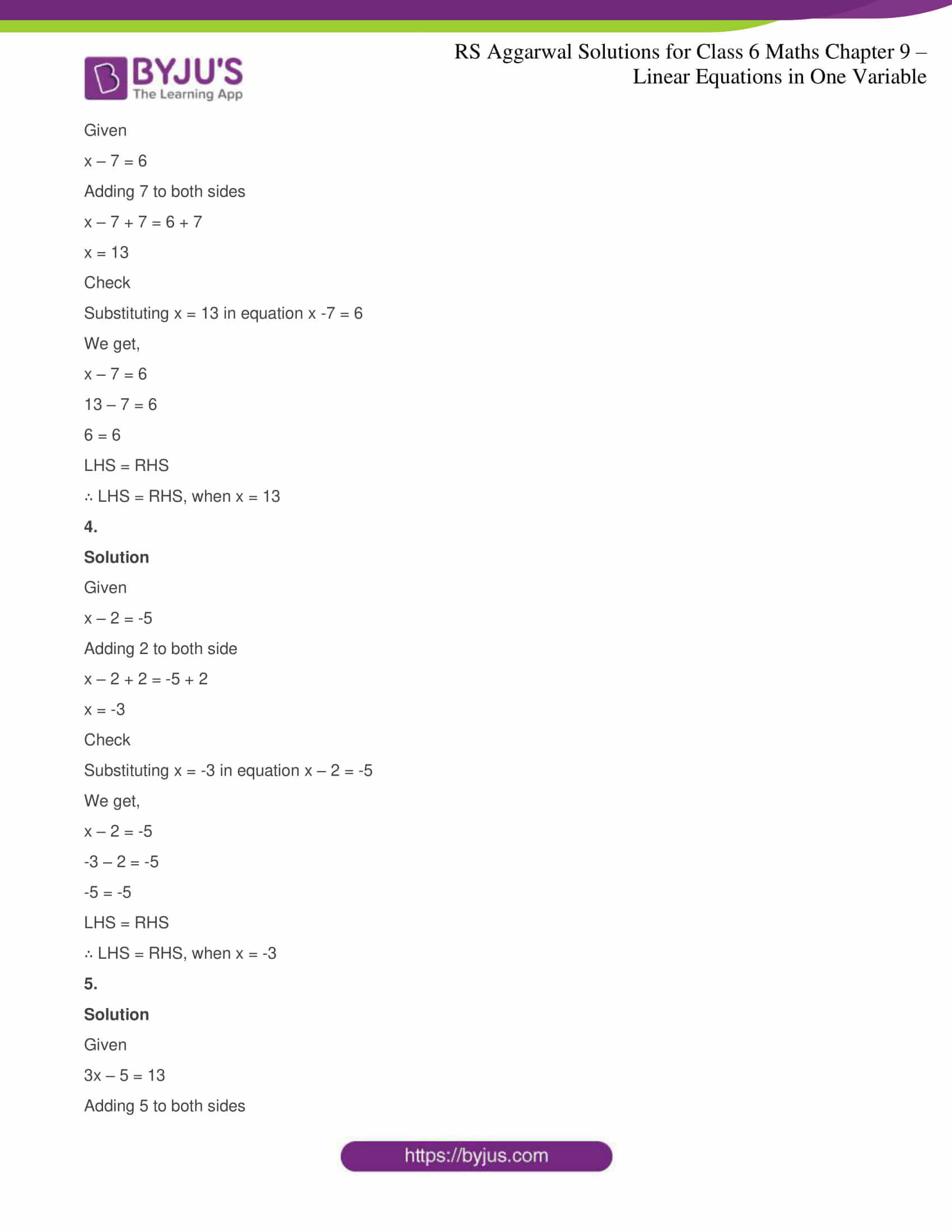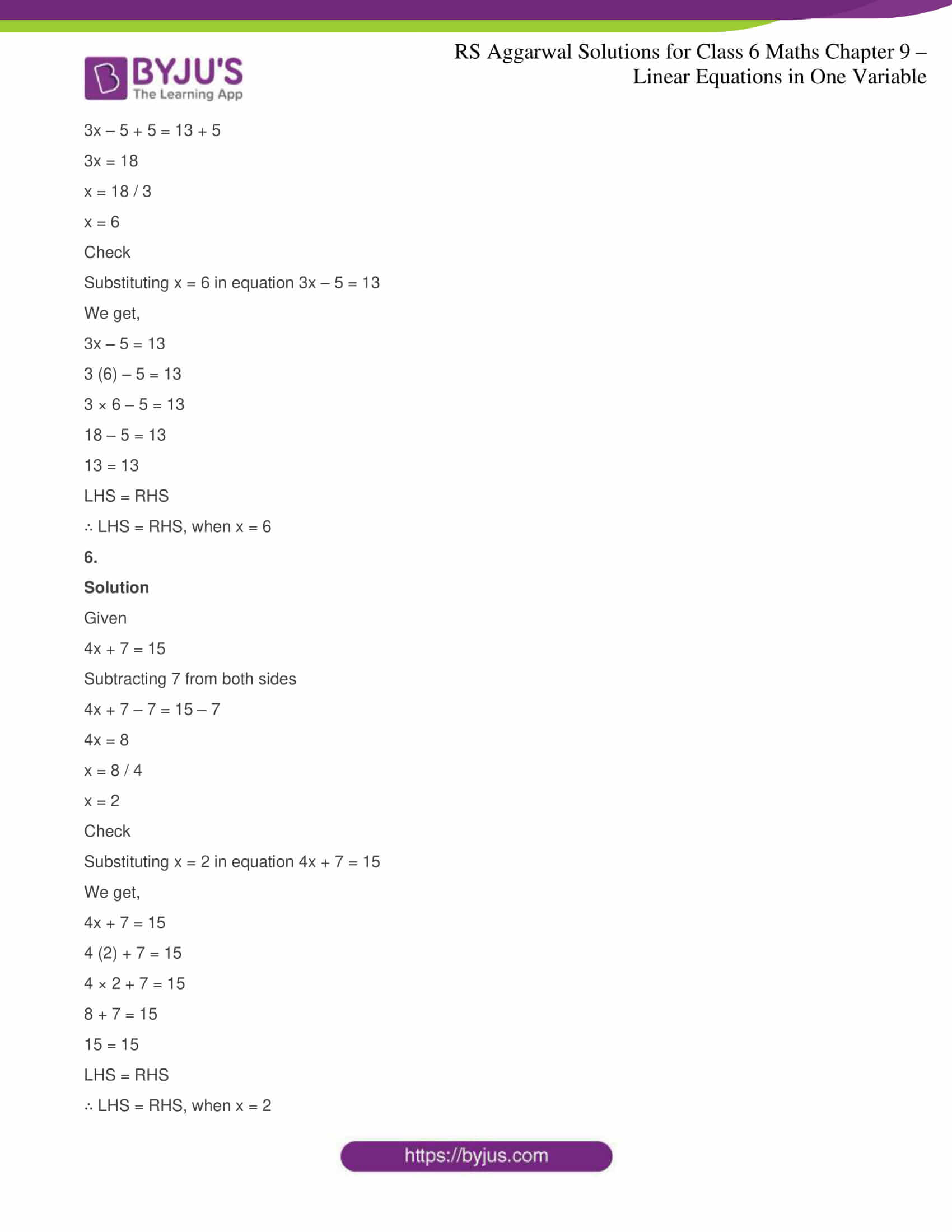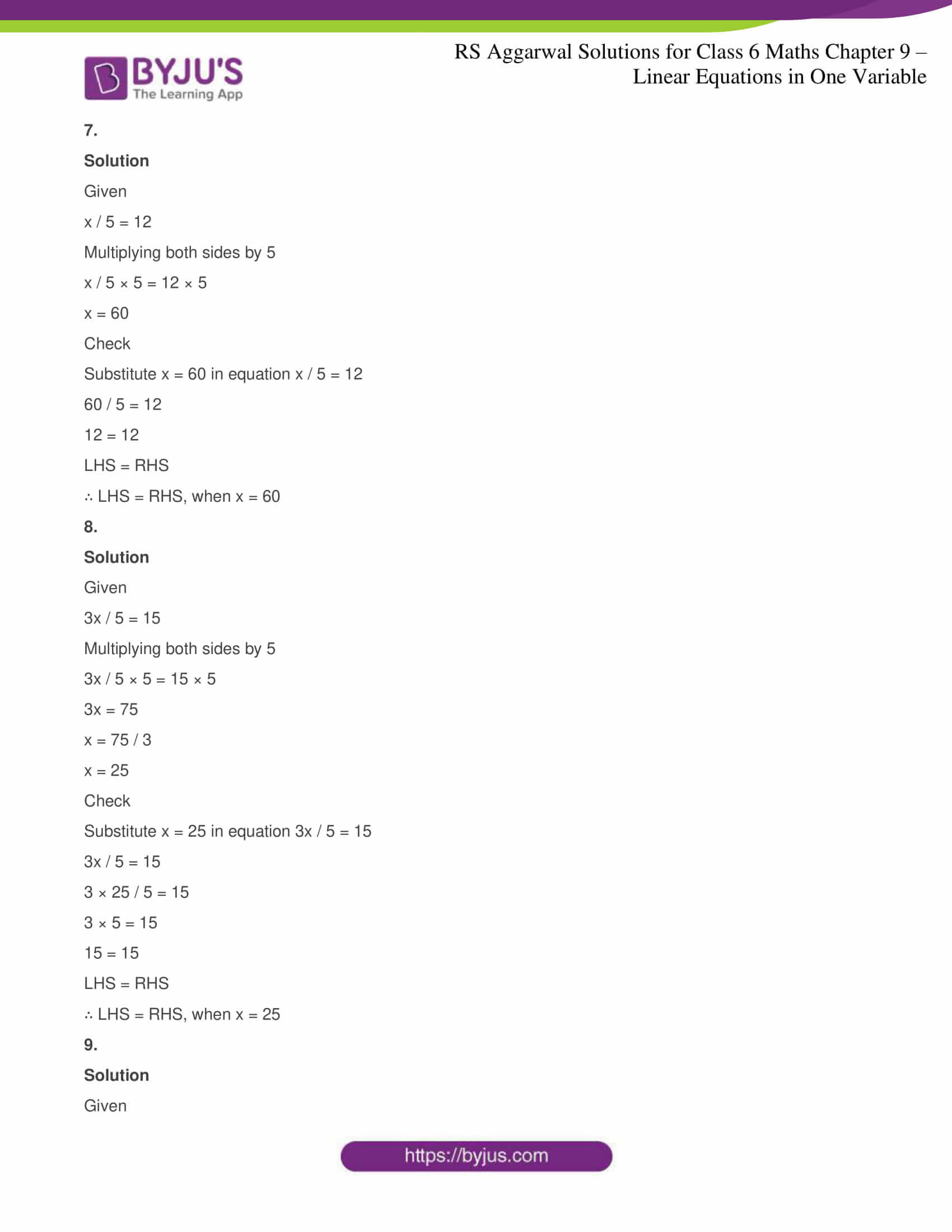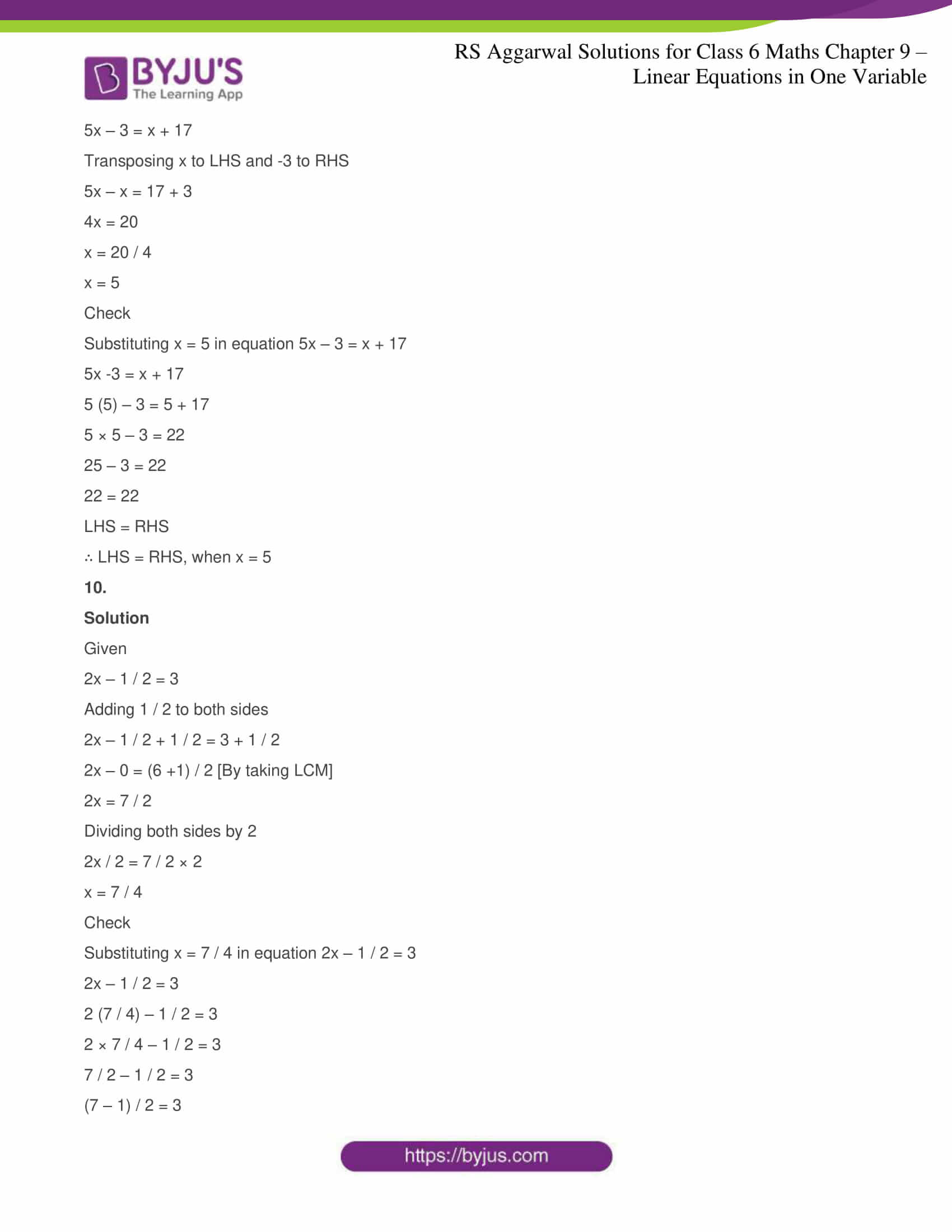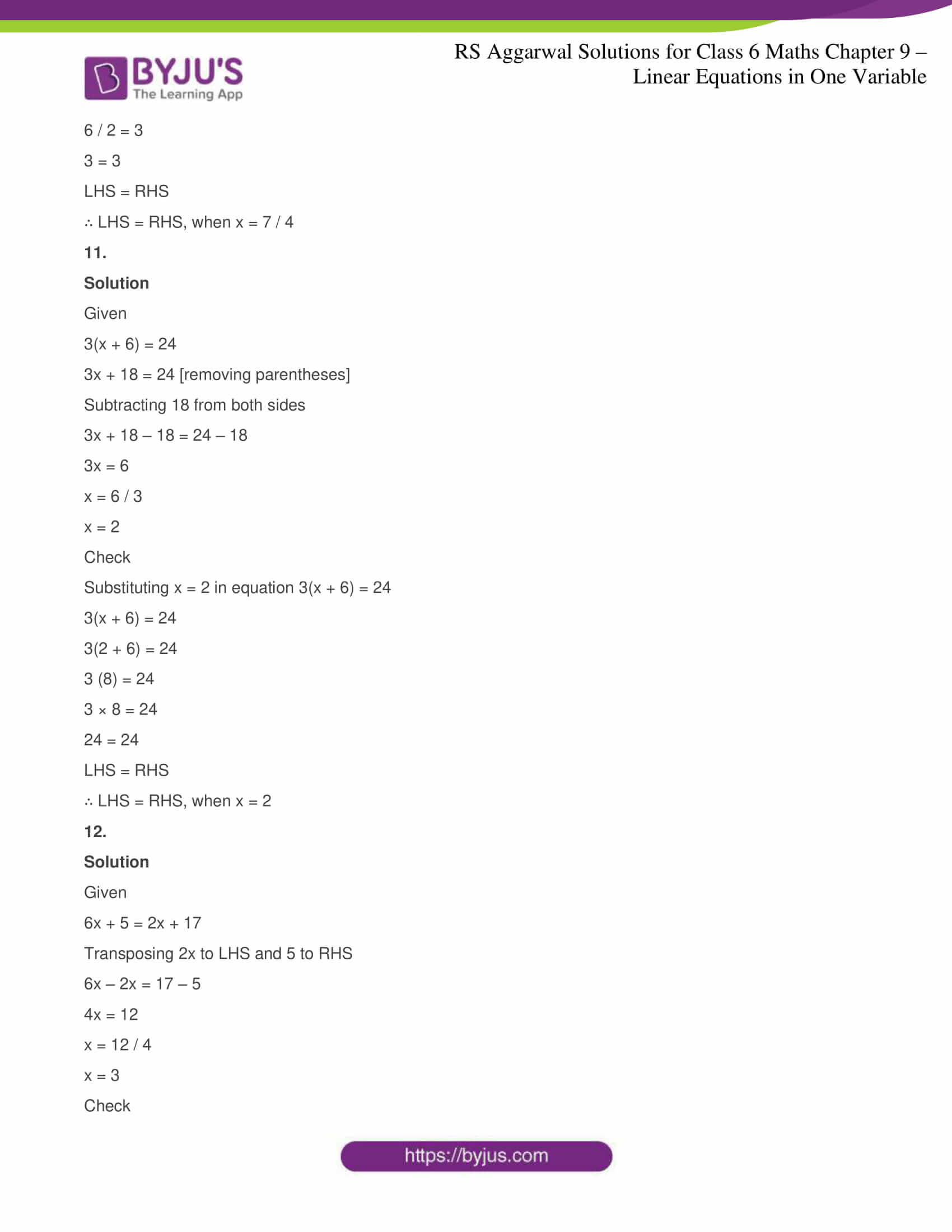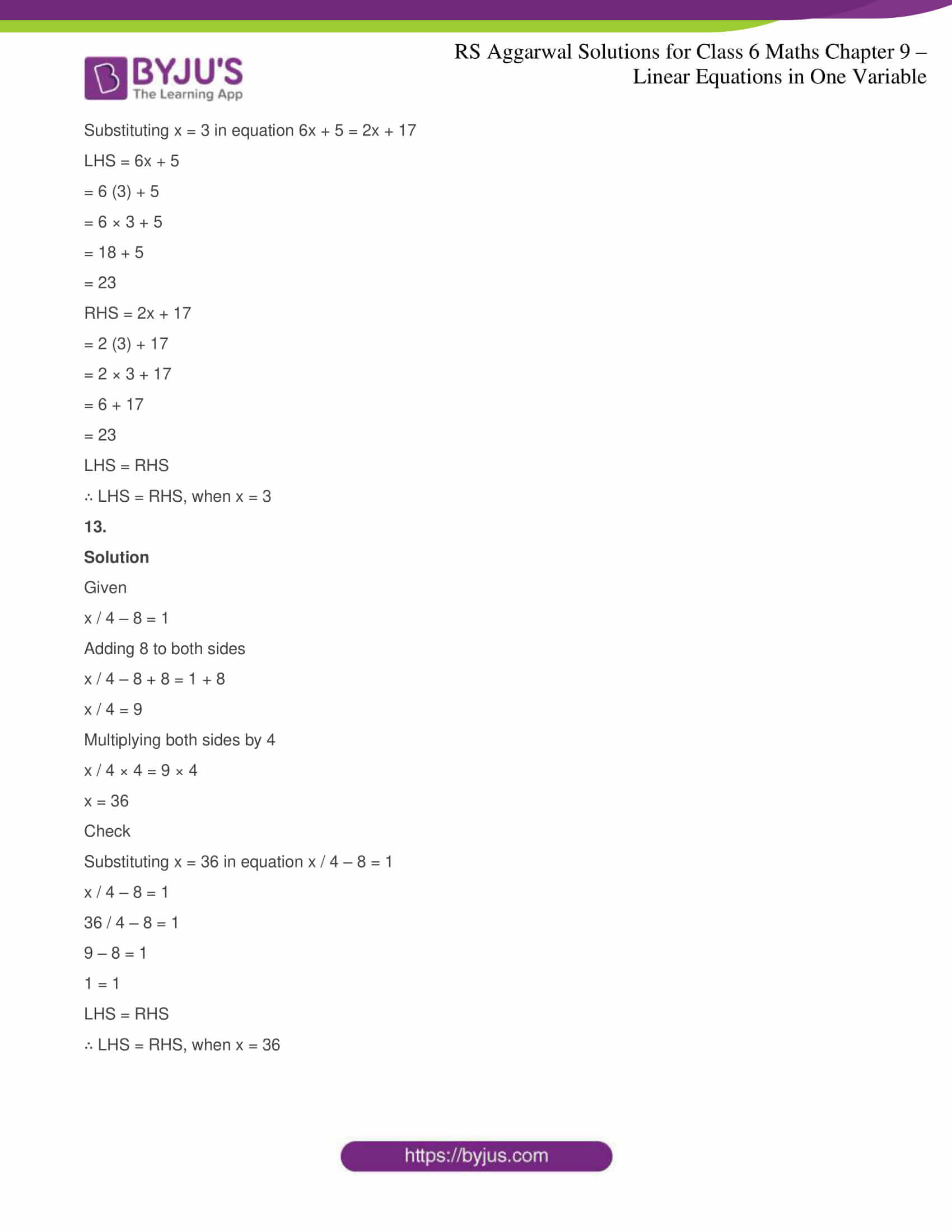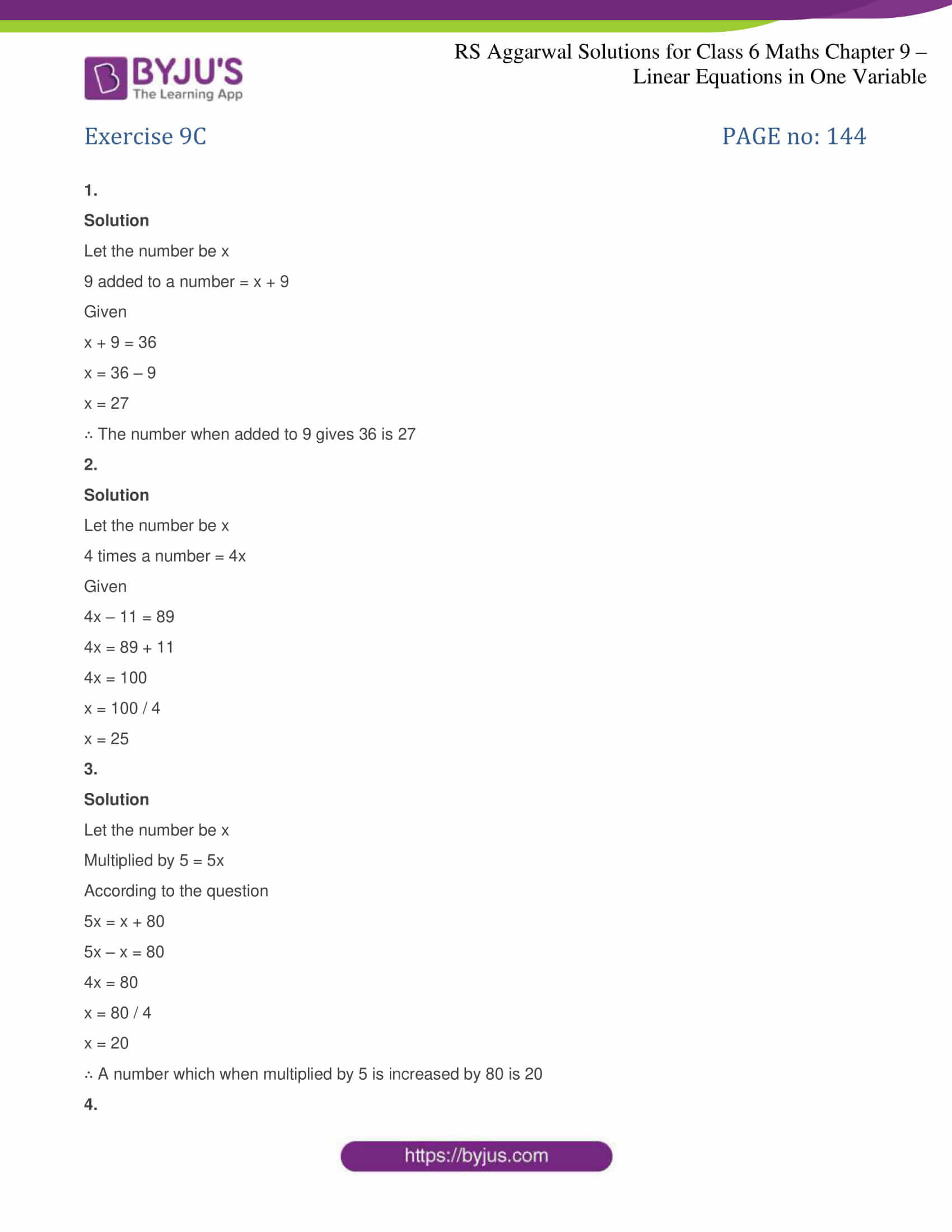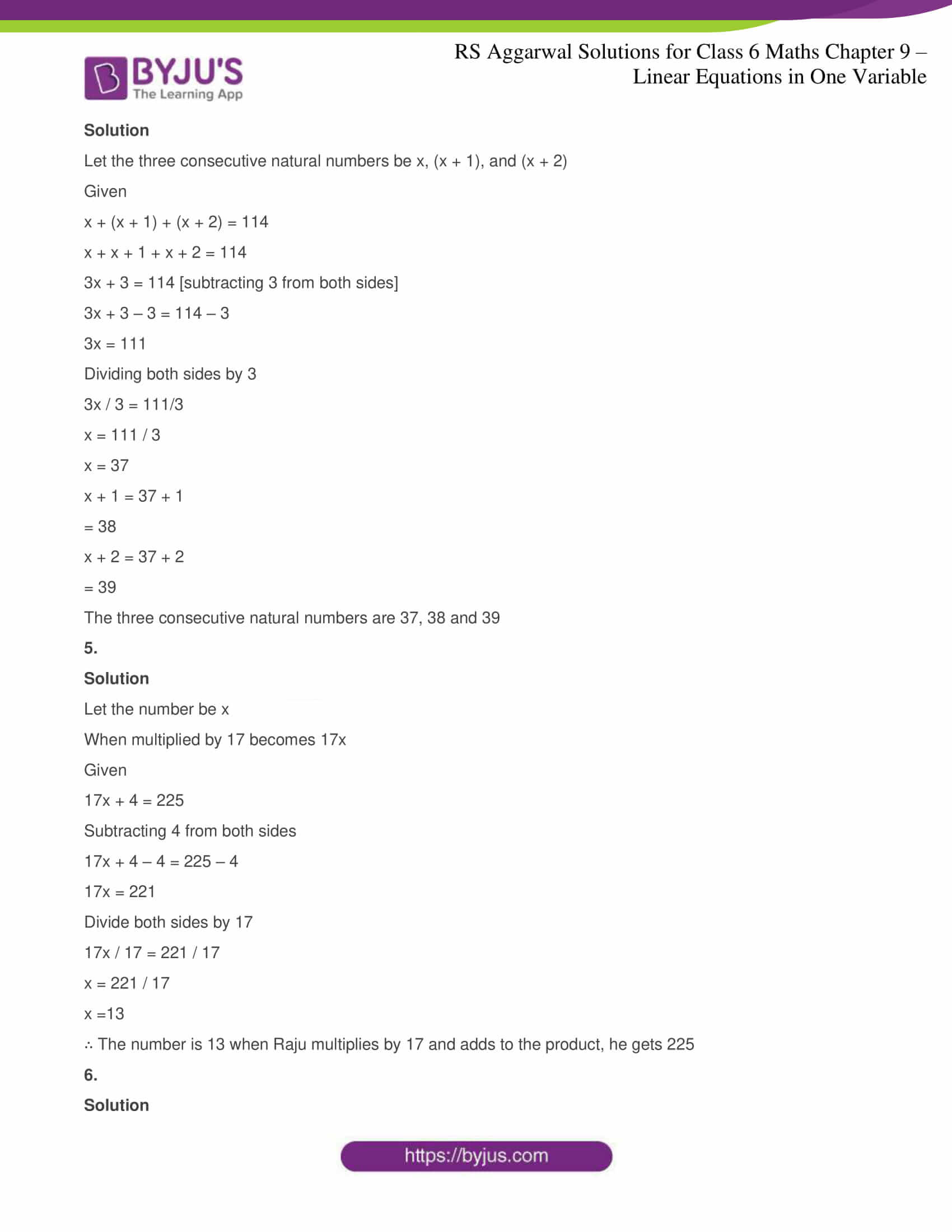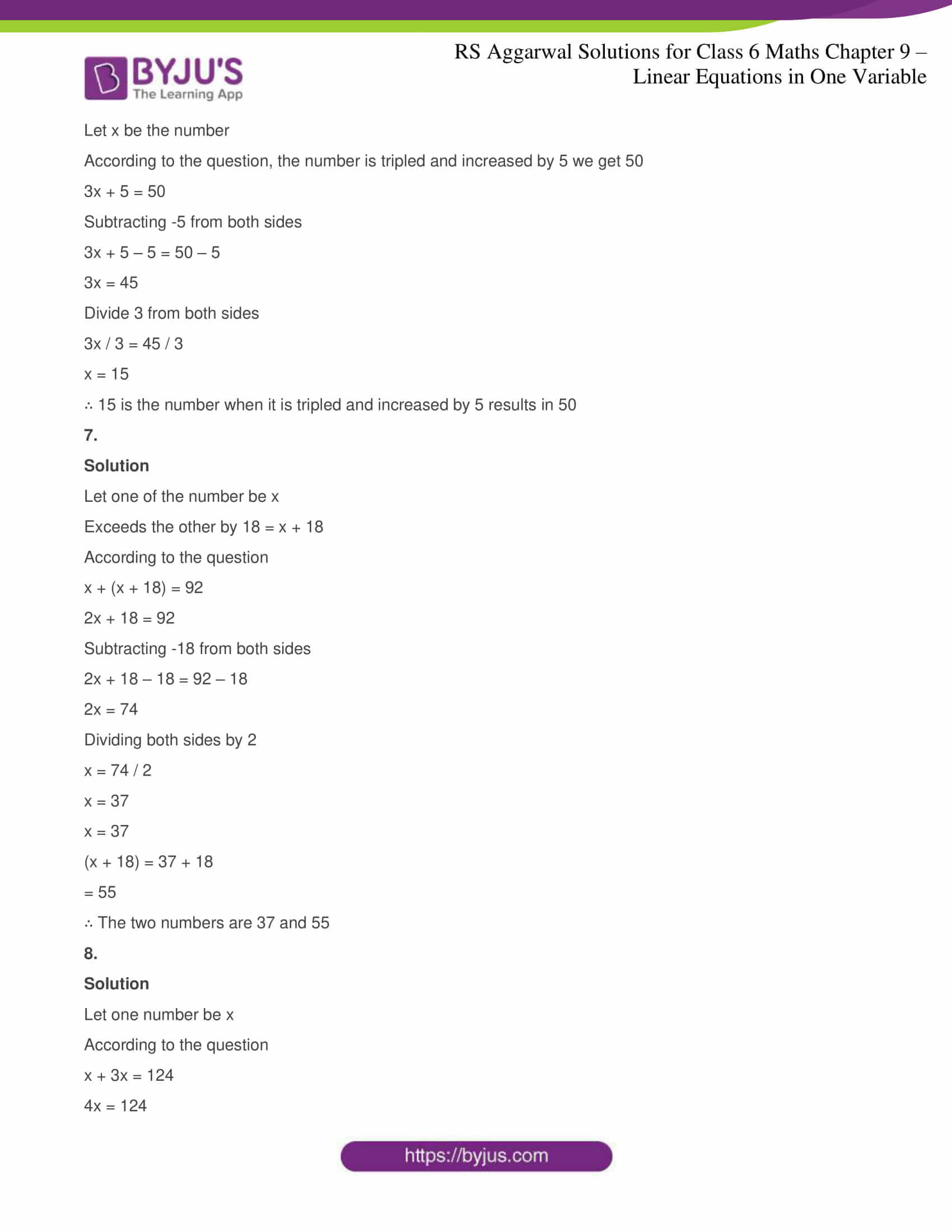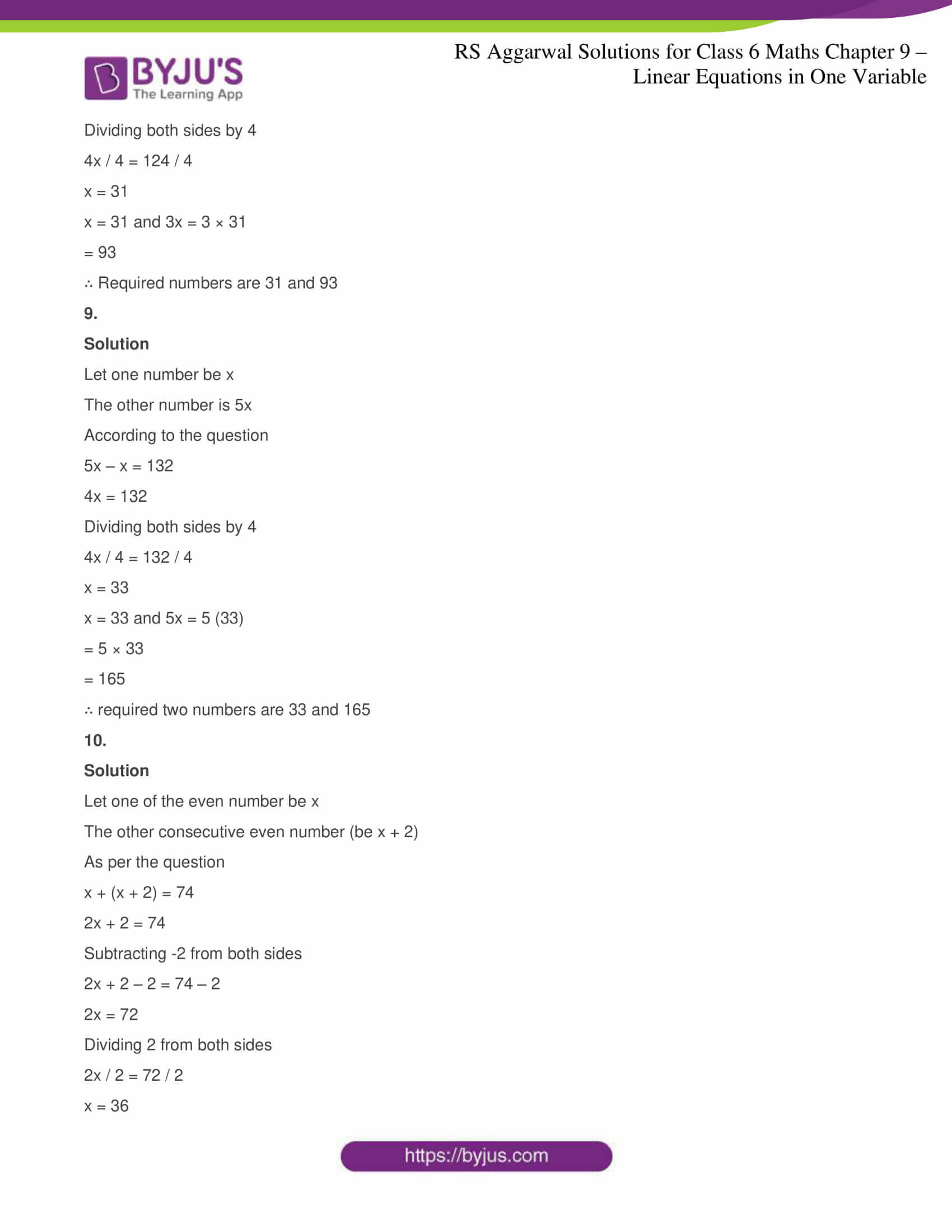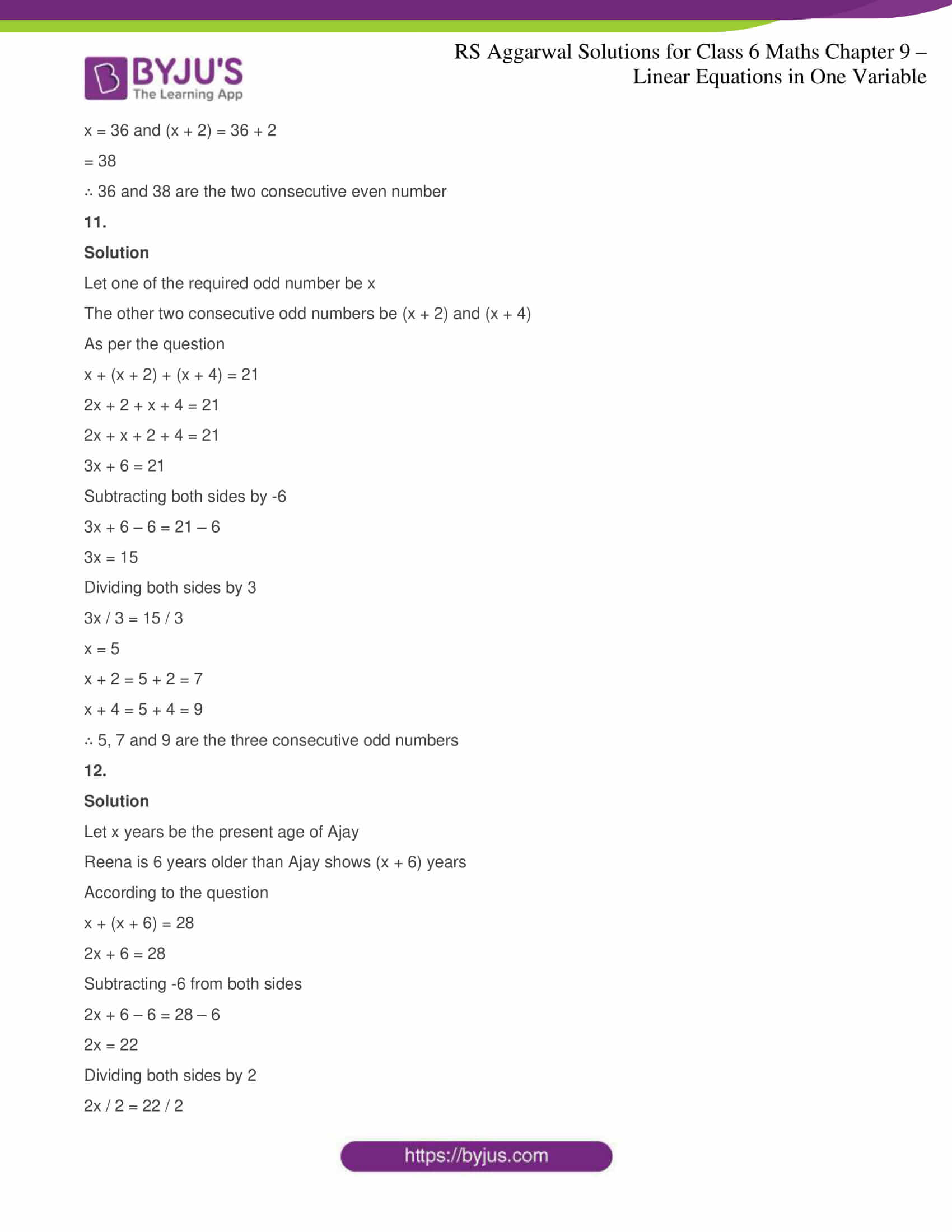### RS Aggarwal Solutions for Class 6 Maths Chapter 9 Linear Equations in One Variable

Chapter 9 – Linear Equations in One Variable consists of 3 exercises. RS Aggarwal Solutions are solved in detail for each question in every exercise. Let’s have a look at the topics which are included in this Chapter

• Systematic method for solving an equation
• Applications of equations

### Chapter Brief of RS Aggarwal Solutions for Class 6 Maths Chapter 9 – Linear Equations in One Variable

A linear equation is an equation in which the highest power of the variables is 1. Variables mentioned in terms of any alphabets such as a, b, c, x, y. There are four rules to solve an equation

(i) We can add the same number to both sides of an equation

(ii) We can subtract the same number from both sides of an equation

(iii) We can multiply both sides of an equation by the same non zero number

(iv) We can divide both sides of an equation by the same non zero number

We can solve linear equations easily by using these rules. They are used for comparing rates of pay, budgeting, making predictions.